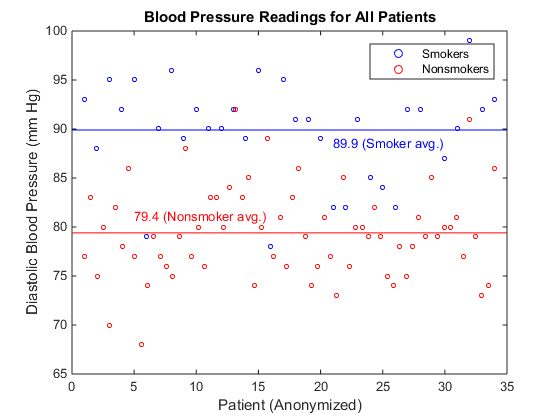## Sort and Plot MATLAB Data from Python

This example shows how to sort data about patients into lists of smokers and nonsmokers in Python® and plot blood pressure readings for the patients with MATLAB®.

Start the engine, and read data about a set of patients into a MATLAB table. MATLAB provides a sample comma-delimited file, `patients.dat`, which contains information on 100 different patients.

```import matlab.engine eng = matlab.engine.start_matlab() eng.eval("T = readtable('patients.dat');",nargout=0) ```

The MATLAB `readtable` function reads the data into a table. The engine does not support the MATLAB table data type. However, with the MATLAB `table2struct` function you can convert the table to a scalar structure, which is a data type the engine does support.

```eng.eval("S = table2struct(T,'ToScalar',true);",nargout=0) eng.eval("disp(S)",nargout=0) ```
``` LastName: {100x1 cell} Gender: {100x1 cell} Age: [100x1 double] Location: {100x1 cell} Height: [100x1 double] Weight: [100x1 double] Smoker: [100x1 double] Systolic: [100x1 double] Diastolic: [100x1 double] SelfAssessedHealthStatus: {100x1 cell} ```

You can pass `S` from the MATLAB workspace into your Python session. The engine converts `S` to a Python dictionary, `D`.

```D = eng.workspace["S"] ```

`S` has fields that contain arrays. The engine converts cell arrays to Python `list` variables, and numeric arrays to MATLAB arrays. Therefore, `D["LastName"]` is of data type `list`, and `D["Age"]` is of data type `matlab.double`.

Sort blood pressure readings into lists of smokers and nonsmokers. In `patients.dat`, the column `Smoker` indicated a smoker with logical 1 (true), and a nonsmoker with a logical 0 (false). Convert `D["Smoker"]` to a `matlab.logical` array for sorting.

`smoker = matlab.logical(D["Smoker"])`

Convert the `Diastolic` blood pressure readings and `Smoker` indicators into 1-by-100 MATLAB arrays for sorting.

```pressure = D["Diastolic"] pressure.reshape((1,100)) pressure = pressure smoker.reshape((1,100)) smoker = smoker ```

Sort the `pressure` array into lists of blood pressure readings for smokers and non-smokers. Python list comprehensions provide a compact method for iterating over sequences. With the Python `zip` function, you can iterate over multiple sequences in a single `for` loop.

```sp = [p for (p,s) in zip(pressure,smoker) if s is True] nsp = [p for (p,s) in zip(pressure,smoker) if s is False] ```

Display the length of `sp`, the blood pressure readings for smokers in a `list`.

```print(len(sp)) ```
```34 ```

Display the length of `nsp`, the `list` of readings for nonsmokers.

```print(len(nsp)) ```
```66 ```

Calculate the mean blood pressure readings for smokers and nonsmokers. Convert `sp` and `nsp` to MATLAB arrays before passing them to the MATLAB `mean` function.

```sp = matlab.double(sp) nsp = matlab.double(nsp) print(eng.mean(sp)) ```
```89.9117647059 ```

Display the mean blood pressure for the nonsmokers.

```print(eng.mean(nsp)) ```
```79.3787878788 ```

Plot blood pressure readings for the smokers and nonsmokers. To define two x-axes for plotting, call the MATLAB `linspace` function. You can plot the 34 smokers and 66 nonsmokers on the same scatter plot.

```sdx = eng.linspace(1.0,34.0,34) nsdx = eng.linspace(1.0,34.0,66) ```

Show the axes boundaries with the `box` function.

```eng.figure(nargout=0) eng.hold("on",nargout=0) eng.box("on",nargout=0) ```

You must call the `figure`, `hold`, and `box` functions with `nargout=0`, because these functions do not return output arguments.

Plot the blood pressure readings for the smokers and nonsmokers, and label the plot. For many MATLAB functions, the engine can return a handle to a MATLAB graphics object. You can store a handle to a MATLAB object in a Python variable, but you cannot manipulate the object properties in Python. You can pass MATLAB objects as input arguments to other MATLAB functions.

```eng.scatter(sdx,sp,10,'blue') ```
```<matlab.object object at 0x22d1510> ```

In the rest of this example, assign the output argument of MATLAB functions to `h` as a placeholder.

```h = eng.scatter(nsdx,nsp,10,'red') h = eng.xlabel("Patient (Anonymized)") h = eng.ylabel("Diastolic Blood Pressure (mm Hg)") h = eng.title("Blood Pressure Readings for All Patients") h = eng.legend("Smokers","Nonsmokers") ```

Draw lines showing the average blood pressure readings for smokers and nonsmokers.

```x = matlab.double([0,35]) y = matlab.double([89.9,89.9]) h = eng.line(x,y,"Color","blue") h = eng.text(21.0,88.5,"89.9 (Smoker avg.)","Color","blue") y = matlab.double([79.4,79.4]) h = eng.line(x,y,"Color","red") h = eng.text(5.0,81.0,"79.4 (Nonsmoker avg.)","Color","red") ```